# Sum facts for kids

Kids Encyclopedia Facts

The sum of two numbers is what we get when we add the two numbers together. This operation is called summation. There are a number of ways of writing sums, with the most common being:

• Addition (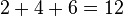$2+4+6 = 12$)
• Summation (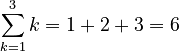$\sum_{k=1}^3 k = 1+2+3=6$)
• Computerization:
Sum = 0
For I = M to N
Sum = Sum + X(I)
Next I (in Visual BASIC)

## Sigma notation

Sigma notation is a mathematical notation to write long sums in a short way. Sigma notation uses the Greek letter Sigma (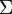$\Sigma$), and takes upper and lower bounds which tell us where the sum begins and where it ends. The lower bound usually has a variable (called the index, often denoted by$i$,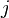$j$ or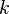$k$) along with a value, such as "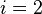$i=2$". This tells us that the summation begins at 2, and goes up by 1 until it reaches the number on the top.

## Properties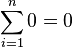$\sum_{i=1}^n 0 = 0$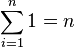$\sum_{i=1}^n 1 = n$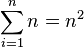$\sum_{i=1}^n n = n^2$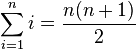$\sum_{i=1}^n i = \frac{n(n+1)}{2}$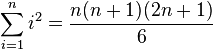$\sum_{i=1}^n i^2 = \frac{n(n+1)(2n+1)}{6}$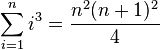$\sum_{i=1}^n i^3 = \frac{n^2 (n+1)^2}{4}$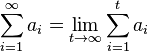$\sum_{i=1}^\infty a_i = \lim_{t \to \infty} \sum_{i=1}^{t} a_i$

## Applications

Sums are used to represent series and sequences. For example: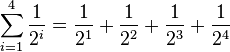$\sum_{i=1}^4 \frac{1}{2^i} = \frac{1}{2^1} + \frac{1}{2^2} + \frac{1}{2^3} + \frac{1}{2^4}$

The geometric series of a repeating decimal can be represented in summation. For example: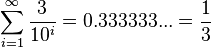$\sum_{i=1}^\infty \frac{3}{10^i} = 0.333333... = \frac{1}{3}$

The concept of an integral is a limit of sums, with the area under a curve being defined as: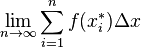$\lim_{n \to \infty} \sum_{i=1}^n f(x_i^*)\Delta x$

## Related pagesSum Facts for Kids. Kiddle Encyclopedia.